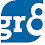# Simple Interest - Practice Problems

Here are some Simple Interest Practice Problems. Before Doing Problems, Have a look at the Basics HERE

1.  Find the Simple Interest on Rs 750 in 4 years at 15% per annum

here S.I =  PTR/100
=  (750 X 4 X 15) / 100 =  450
2. On what sum of money will the Simple Interest be Rs. 2000 in 5 years at 8% Per Annum?

So, P = 100I / TR
=  (100X2000) / 40  = 5000

3. A man invested Rs. 20,000 at 10% P.A. Rs. 15,000 at 12% P.A. and some money at 15% P.A. If the total annual interest recieved is Rs. 56,00, find the money invested by him at 15% P.A
here, the total Interest he recieved is Rs. 56,00.
So,  find the total interest and try to equate it with 56,00

So, the total interest = Σ [ (PTR)/100 ]    [ here Sigma symbol ( Σ ) represents the Total]

= 200X10+150X12+ (P/100)X15 = 5600

3800+ (3P/20) = 5600

= 3P/20  =  1800 => P = 12000/-

4. On a sum of money the rate of interest is 5% Per Annum for the first 3 years, 6% Per Annum for the next 4 years, and 8% Per Annum for the next years beyond the first 7 Years. If the interest obtained in 12 Years is Rs. 3,950, Find the Sum?

5% X 3 + 6% X 4 + 8% X 5
=  15% + 24% + 40% = 79%
=>  (79/100) P  = 3950

=> P  =  (39500/79)  =  5000

5. A sum was put at 5% at a certain rate for 5 Years. Had it been put at 3% Per Annum higher rate, it would have fetched Rs. 900 more. Find the Sum?

P =   100I / TR      [Note : Here I is Additional Interest and R is additional Rate]

= (100 X 900) / (5X3)
= 6000

6. On a certain Sum of Money the Simple Interest in 2 years at 10% P.A is Rs. 125, what would be the S.I if the rate of Interest will be 12% P.A in 3 Years?

Here the Principle is same for both the cases. I mean  p = P

=>    100i / tr  =  100I / TR

=  125 / (2 x 10)  =  I / (3 x 12)

= >  I = 225

7. The S.I on Rs 6400 at 12 1/2 % per annum is Rs. 2000. Find the Period ?

T = 100I / PR   =  (100 X 2000 X 2) / (6400 X 25)  =  2 1/2 years

8. The S.I on Rs. 6000 in 3 Years and 4 Months is Rs. 3000. Find the rate percent Per Annum ?

[ here T = 3 4/12 = 3 1/3  = 10/3 years]

I = PTR / 100 =>  R = 100I / PT

=>  (100 X 3000 X 3) / (6000 X 10 ) = 15

9. Manish took a loan of Rs. 4000 at S.I. After 2 Years he cleared the loan by paying Rs. 5600. Find the Rate % P.A?

[ Here Manish took Rs. 4000 and Payed 5600. So the Interest will be 5600-4000 = 1600 ]

So, R = 100I / PT

=  (100 X 1600) / (400 X 2)

=  20

10. A lent Rs. 25000 to B for 4 years and Rs. 40,000 to C for 3 1/2 years and got Rs. 24,000 S.I from both B and C. Find the rate PCPA

Σ [ (PTR)/100 ]   =  250 X 4R = 400 X (7/2) R =  2400R

=> R = 10

Check the Basics of Compound Interest HERE

1.in question 3 the intrest is 56000/- and u have wrongly calculated with 5600/-

1.i guess u r wrong

2.1sol: 15%pa => for 1yr 15%
4yrs =>(4*15=60)
so 60% on principal ie 750
=(60/100)*750
=450

3.2000 5 8%
2sol:
1yr =>8%
5yr =40%
40% => 2000
100% =>(2000*100)/40
=5000
(SINCE WE ASSUME PRINCIPAL =100 SO CALCULATED FOR 100%)

4.6SOL:
1yr => 10%
2yrs => 20%
now given 125rs SI for 2 yrs
so 20% => 125
100% =>?
hence p =(125*100)/20 =625
now, given 1yr => 12% so for 3yrs 36%
hence SI= 36% of 625=225

5.SI and CI is too confusing even after seeing the basics and problems.
Pl avoid 5600 instead of 56000.
Already can't understand anything and if its like this then that's all, null:(

1.Sorry for the inconvenience friend. Its a typing mistake, removed the additional Zero now. Thanks for the update :)

2.awesome sir...... ur website is, simply i can say is ...marvalleous. go on... thanks a lottttttttttttttttttt. love u all who are running this website

6.dont short te calculations mam...it leads to confusion..let it be clear so that we may understand better.

1.Ok friend. We will try to post in detailed steps soon.

Thanks for the suggestion.

7.thanks ................

8.many many thanx

9.There is mistake in 5th solution..I=900,u have written I=90..Plz correct it..others would confuse...

10.Hi comment box,I miss u nowadays.

11.As per 7 Question:
The Formula be T= 100*i/ PR
=> 100*2400/6400*25
=> 240000/160000
=> 1.5 Years (Period)

How did you get 100*2400*(2)<-- Reply will be appreciated.

12.solve some more problems.it will be helped to better understan

13.10th one problem is more confusing me.. try to explain briefly ,,,,

14.Rohan lends 1000 in four parts. If he gets 8% interest on 2000, 7 1/2% on 4000 and 8 1/2% on 1400 what percent of interest must he get for the remainder if his average annual interest is 8.13%?

plz can anyone xplian this problem?

15.9th solution
((1600*100)/(4000*2))=20. Not 400

16.Q7 Solution is 5/2